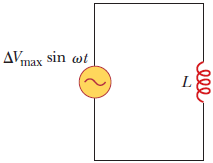# Problem: In a purely inductive AC circuit as shown in the figure, ΔVmax = 100 V.The maximum current is 8.00 A at 80.0 Hz. Calculate the inductance L.

###### FREE Expert Solution

Inductive reactance:

$\overline{){{\mathbf{X}}}_{{\mathbf{L}}}{\mathbf{=}}{\mathbf{\omega }}{\mathbf{L}}}$

Ohm's law:

$\overline{){\mathbf{V}}{\mathbf{=}}{\mathbf{i}}{\mathbf{R}}}$

Angular frequency:

$\overline{){\mathbf{\omega }}{\mathbf{=}}{\mathbf{2}}{\mathbf{\pi }}{\mathbf{f}}}$

83% (382 ratings)###### Problem Details

In a purely inductive AC circuit as shown in the figure, ΔVmax = 100 V.The maximum current is 8.00 A at 80.0 Hz. Calculate the inductance L.

Frequently Asked Questions

What scientific concept do you need to know in order to solve this problem?

Our tutors have indicated that to solve this problem you will need to apply the Inductors concept. You can view video lessons to learn Inductors. Or if you need more Inductors practice, you can also practice Inductors practice problems.